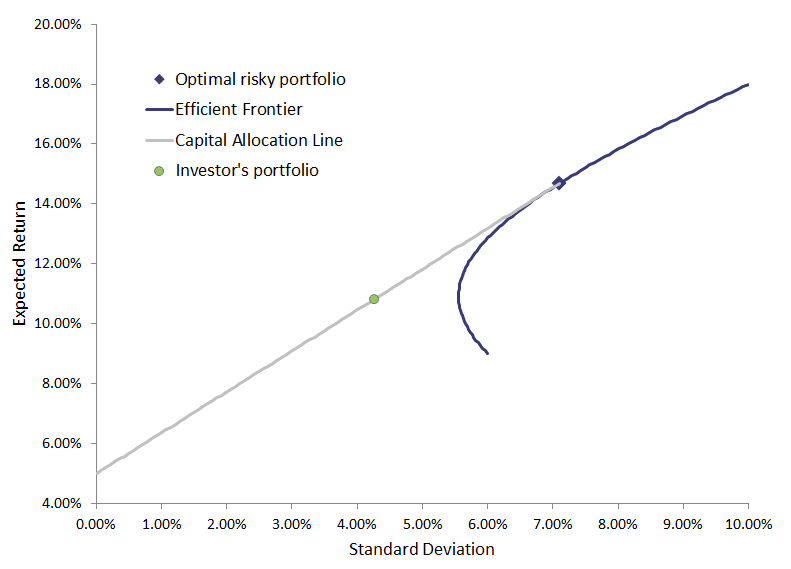# Portfolio optimizaton

Now we combine the insights we gained from constructing the capital allocation line and the set of efficient portfolios using Markowitz’s portfolio selection approach. Combining both elements allows us to perform portfolio optimization to determine the optimal risky portfolio.

But let’s first recapitulate what we’ve learned so far. From the construction of the capital allocation line (CAL), we have learned that the steeper the CAL, the more attractive the portfolios we can invest in. This is because portfolios on a steeper CAL compensate the investor more for taking additional risk. They have a higher slope and thus Sharpe ratio. From Markowitz’s portfolio selection approach, we determined a set of portfolios that provides the best risk/return trade-off for a given level of risk.

## Mean-variance optimizaton in practice

Portfolio optimization allows us to select the portfolio on the efficient frontier with the highest Sharpe ratio. This is where the capital allocation line is tangent (i.e. just touches) to the efficient frontier.  The figure below illustrates how we combine the CAL and the efficient frontier to determine the optimal risky portfolio.This particular portfolio (highlighted here with a square) will serve as the risky asset in the investor’s overall portfolio.

The investor should still decide how much of his portfolio to invest in the risky portfolio we just determined, and how much to invest in the riskfree asset. This subjective decision depends on how much risk the investor is willing to take. For example, an investor could decide to invest 40% in the riskfree asset, and 60% in the optimal risky portfolio. This particular portfolio corresponds to the green dot on the capital allocation line.

## Using Excel

The Excel file available on at the bottom of this page allows you to implement the entire portfolio optimization we just described above.

## Limitations of portfolio optimization

There are also limitations to the above portfolio optimization approach.

• First, we need to estimate a lot of variables to be able to estimate Markowitz’s model. As an example, suppose we want to determine the optimal risky portfolio and have 50 securities we want to include. In that case, we need to estimate 50 variances, 50 expected returns, and (502-50)/2 = 1125 correlation coefficients.
• Second, the results of the portfolio optimization method proposed by Markowitz is very sensitive to the outputs. When the expected returns, standard deviation, and correlations change slightly, the weights we obtain typically vary a lot. Third, the weights we obtain are often extreme. More often than not, the approach suggests we should allocate everything in just a hand full of shares.

These limitations lead some people to prefer alternative portfolio construction approaches.

## Summary

When we combine the capital allocation line and markowitz’s portfolio selection approach, we can perform portfolio optimization.

### Portfolio optimization

Need to have more insights? Download our free excel file: Portfolio optimization.